## Monday, November 23, 2009

### Off on a Tangent - The Heptagon and The Savonnerie CarpetThe Savonnerie Carpet is in Mr. Deering's Sitting Room. We are planning several products featuring this beautiful carpet.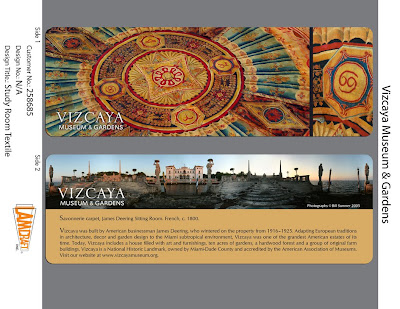The carpet is 28 feet across and it's very difficult to get a straight on photo. As I was studying the detail to create a direct image I realized the polygons with the zodiac detail were 7 sided heptagons.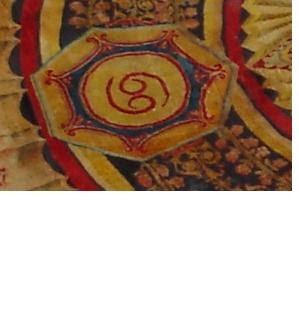I pulled out my trusty templates knowing there is no way to free hand a heptagon and there were only 6 sided hexagons. So, I went to Google Images to create my own template.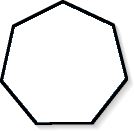I am off on another treasure hunt. First I find http://origami.oschene.com/archives/2006/10/01/how-to-make-a-heptagon-from-a-circle/ I am amazed at the minds that ponder how to origami a heptagon. My daughter laughed every time I asked the 5 stores we went to if they had a heptagon template. Now I have to figure out how to create an origami heptagon.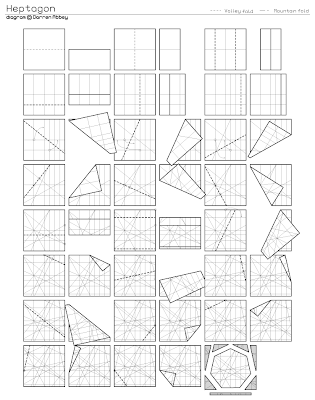Then I found the instructions for the heptagon. I am set on that front, now back to my template. http://www.ganymeta.org/~darren/origamishapes.php?shape=heptagonBut wait this is an interesting image. http://intermath.coe.uga.edu/dictnary/everyday.asp?termid=165 "The emblem is called the Sigil of Ameth. It was created in the 16th century for Queen Elizabeth I by Dr. John Dee, an advisor and astrologer. A regular heptagon, or a seven-sided polygon lies directly inside the outer circle."

So is there a connection between the heptagon, astrology, and the zodiac? While I could find mystical relations to the 7 pointed star I did not find a direct relation to the zodiac. http://wikicompany.org/wiki/911:Occult_symbolism_III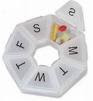While I am out on this tangent there was a lot of medieval superstition about the number 7. I will leave you with this link to ponder for yourself the mysteries of life and the number 7 while I go bake Thanksgiving pies. Look for the new bookmarks next month.

The Heptagram and the Properties of Seven

The number seven has some curious particularities. For example 1 divided by 7 gives a simple recurring decimal of six digits:

0.142857142857142857...

and this one multiplied successively by 2, 3, 4, 5, 6, gives products where we find the same numbers in the same order:

142857 x 2 = 285714 142857 x 3 = 428571 142857 x 4 = 571428 142857 x 5 = 714285 142857 x 6 = 857142

For the ancients, seven was known as "the virgin", as no number less than seven divides into it. For example, two divides four, six, eight, and ten; three divides six and nine; four divides eight; five divides ten; six divides six. But no number divides seven evenly. Thus seven is a "virgin", as no other number enters into it, symbolic of its numeric purity.

Among all prime numbers, seven is the first number that does not enter in the Euclidean circle. Dividing all other root numbers into 360 results in a whole number, except for seven, as follows:

360 / 1 = 360 360 / 2 = 180 360 / 3 = 120 360 / 4 = 90 360 / 5 = 72 360 / 6 = 60 360 / 7 = 51.42857143 etc., repeating irrationally. 360 / 8 = 45 360 / 9 = 40

Symbolically, this means that the heptad (seven) defies precise quantification, much as with the values of Pi (circle) and Phi (Golden Mean Ratio). Put another way, the heptad cannot be measured, or defined or captured, by linear rationalism.

The heptagon is the only basic geometric form that cannot be created via the Vesica Pisces, which is often regarded by sacred geometry as the "womb" of existence. It is because of this unique form that the value of seven is regarded as virginal, because it is not "born" through the geometric and arithmetic precision that the other root numbers are.

The steeper angle of the heptagram is 51.428* degrees. This is very close to the angle of slope of the sole surviving Wonder of the ancient world, the Great Pyramid of Giza, Egypt, which is about 51.8 degrees. Further, the lesser slope of the heptagram is essentially the same as the slope of the internal passageways in the Great Pyramid, about 26.5 degrees. If a double heptagram (fourteen point star) is constructed, then the form of the Great Pyramid is found in the center of interfacing lines, with a 51.428 degree angle of slope. Many ancient Greek temples (such as those dedicated to Athena, Minerva, and Neith) were based on seven-fold geometry. All this is suggestive of the importance ancient architects attached to this number seven.